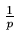Next: 3.9.1 Analysis of Expected Search Cost Up: 3. Dictionaries Previous: 3.8.1 Initialization:

# 3.9 Analysis of Skip Lists

In a skiplist of 16 elements, we may have

• 9 elements at level 1
• 3 elements at level 2
• 3 elements at level 3
• 1 element at level 6

• One important question is:
Where do we start our search? Analysis shows we should start from level L(n) where

L(n) = log2n

In general if p is the probability fraction,

L(n) = logn

where p is the fraction of the nodes with level i pointers which also have level (i + 1) pointers.

• However, starting at the highest level does not alter the efficiency in a significant way.

• Another important question to ask is:
What should be MaxLevel? A good choice is

MaxLevel = L(N) = logN

where N is an upperbound on the number of elements is a skiplist.

• Complexity of search, delete, insert is dominated by the time required to search for the appropriate element. This in turn is proportional to the length of the search path. This is determined by the pattern in which elements with different levels appear as we traverse the list.

• Insert and delete involve additional cost proportional to the level of the node being inserted or deleted.Next: 3.9.1 Analysis of Expected Search Cost Up: 3. Dictionaries Previous: 3.8.1 Initialization:
eEL,CSA_Dept,IISc,Bangalore